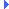FD.io VPP  v19.01.3-6-g70449b9b9 Vector Packet Processing
svm_fifo_segment.h File ReferenceInclude dependency graph for svm_fifo_segment.h:This graph shows which files directly or indirectly include this file:

Go to the source code of this file.

## Data Structures

struct  svm_fifo_segment_private_t

struct  svm_fifo_segment_main_t

struct  svm_fifo_segment_create_args_t

## Macros

#define FIFO_SEGMENT_MIN_FIFO_SIZE   4096

#define FIFO_SEGMENT_MAX_FIFO_SIZE   (2 << 30) /* 2GB max fifo size */

#define FIFO_SEGMENT_ALLOC_CHUNK_SIZE   32 /* Allocation quantum */

#define FIFO_SEGMENT_F_IS_PREALLOCATED   (1 << 0)

#define FIFO_SEGMENT_F_WILL_DELETE   (1 << 1)

#define svm_fifo_segment_flags(_seg)   _seg->h->flags

## Enumerations

enum  svm_fifo_segment_freelist_t { FIFO_SEGMENT_FREELIST_NONE = -1, FIFO_SEGMENT_RX_FREELIST = 0, FIFO_SEGMENT_TX_FREELIST, FIFO_SEGMENT_N_FREELISTS }

## Functions

static svm_fifo_segment_private_tsvm_fifo_segment_get_segment (svm_fifo_segment_main_t *sm, u32 segment_index)

static u8 svm_fifo_segment_has_fifos (svm_fifo_segment_private_t *fifo_segment)

static svm_fifo_tsvm_fifo_segment_get_fifo_list (svm_fifo_segment_private_t *fifo_segment)

int svm_fifo_segment_init (svm_fifo_segment_private_t *s)
Initialize svm fifo segment shared header. More...

int svm_fifo_segment_create (svm_fifo_segment_main_t *sm, svm_fifo_segment_create_args_t *a)
Create an svm fifo segment and initialize as master. More...

int svm_fifo_segment_create_process_private (svm_fifo_segment_main_t *sm, svm_fifo_segment_create_args_t *)
Create an svm fifo segment in process-private memory. More...

void svm_fifo_segment_preallocate_fifo_pairs (svm_fifo_segment_private_t *s, u32 rx_fifo_size, u32 tx_fifo_size, u32 *n_fifo_pairs)
Pre-allocates fifo pairs in fifo segment. More...

int svm_fifo_segment_attach (svm_fifo_segment_main_t *sm, svm_fifo_segment_create_args_t *a)
Attach as slave to an svm fifo segment. More...

void svm_fifo_segment_delete (svm_fifo_segment_main_t *sm, svm_fifo_segment_private_t *s)

svm_fifo_tsvm_fifo_segment_alloc_fifo (svm_fifo_segment_private_t *s, u32 data_size_in_bytes, svm_fifo_segment_freelist_t index)
Allocate fifo in svm segment. More...

void svm_fifo_segment_free_fifo (svm_fifo_segment_private_t *s, svm_fifo_t *f, svm_fifo_segment_freelist_t index)

void svm_fifo_segment_main_init (svm_fifo_segment_main_t *sm, u64 baseva, u32 timeout_in_seconds)

u32 svm_fifo_segment_index (svm_fifo_segment_main_t *sm, svm_fifo_segment_private_t *s)

u32 svm_fifo_segment_num_fifos (svm_fifo_segment_private_t *fifo_segment)
Get number of active fifos. More...

u32 svm_fifo_segment_num_free_fifos (svm_fifo_segment_private_t *fifo_segment, u32 fifo_size_in_bytes)

void svm_fifo_segment_info (svm_fifo_segment_private_t *seg, char **address, size_t *size)

svm_fifo_segment_private_tsvm_fifo_segment_segments_pool (svm_fifo_segment_main_t *sm)
Retrieve svm segments pool. More...

## Variables

format_function_t format_svm_fifo_segment

format_function_t format_svm_fifo_segment_type

## ◆ FIFO_SEGMENT_ALLOC_CHUNK_SIZE

 #define FIFO_SEGMENT_ALLOC_CHUNK_SIZE   32 /* Allocation quantum */

Definition at line 32 of file svm_fifo_segment.h.

## ◆ FIFO_SEGMENT_F_IS_PREALLOCATED

 #define FIFO_SEGMENT_F_IS_PREALLOCATED   (1 << 0)

Definition at line 34 of file svm_fifo_segment.h.

## ◆ FIFO_SEGMENT_F_WILL_DELETE

 #define FIFO_SEGMENT_F_WILL_DELETE   (1 << 1)

Definition at line 35 of file svm_fifo_segment.h.

## ◆ FIFO_SEGMENT_MAX_FIFO_SIZE

 #define FIFO_SEGMENT_MAX_FIFO_SIZE   (2 << 30) /* 2GB max fifo size */

Definition at line 31 of file svm_fifo_segment.h.

## ◆ FIFO_SEGMENT_MIN_FIFO_SIZE

 #define FIFO_SEGMENT_MIN_FIFO_SIZE   4096

Definition at line 30 of file svm_fifo_segment.h.

## ◆ svm_fifo_segment_flags

 #define svm_fifo_segment_flags ( _seg ) _seg->h->flags

Definition at line 71 of file svm_fifo_segment.h.

## ◆ svm_fifo_segment_freelist_t

Enumerator
FIFO_SEGMENT_FREELIST_NONE
FIFO_SEGMENT_RX_FREELIST
FIFO_SEGMENT_TX_FREELIST
FIFO_SEGMENT_N_FREELISTS

Definition at line 22 of file svm_fifo_segment.h.

## ◆ svm_fifo_segment_alloc_fifo()

 svm_fifo_t* svm_fifo_segment_alloc_fifo ( svm_fifo_segment_private_t * s, u32 data_size_in_bytes, svm_fifo_segment_freelist_t index )

Allocate fifo in svm segment.

Definition at line 337 of file svm_fifo_segment.c.Here is the call graph for this function:Here is the caller graph for this function:

## ◆ svm_fifo_segment_attach()

 int svm_fifo_segment_attach ( svm_fifo_segment_main_t * sm, svm_fifo_segment_create_args_t * a )

Attach as slave to an svm fifo segment.

Definition at line 292 of file svm_fifo_segment.c.Here is the call graph for this function:Here is the caller graph for this function:

## ◆ svm_fifo_segment_create()

 int svm_fifo_segment_create ( svm_fifo_segment_main_t * sm, svm_fifo_segment_create_args_t * a )

Create an svm fifo segment and initialize as master.

Definition at line 204 of file svm_fifo_segment.c.Here is the call graph for this function:Here is the caller graph for this function:

## ◆ svm_fifo_segment_create_process_private()

 int svm_fifo_segment_create_process_private ( svm_fifo_segment_main_t * sm, svm_fifo_segment_create_args_t * )

Create an svm fifo segment in process-private memory.

Definition at line 238 of file svm_fifo_segment.c.Here is the call graph for this function:Here is the caller graph for this function:

## ◆ svm_fifo_segment_delete()

 void svm_fifo_segment_delete ( svm_fifo_segment_main_t * sm, svm_fifo_segment_private_t * s )

Definition at line 325 of file svm_fifo_segment.c.Here is the call graph for this function:Here is the caller graph for this function:

## ◆ svm_fifo_segment_free_fifo()

 void svm_fifo_segment_free_fifo ( svm_fifo_segment_private_t * s, svm_fifo_t * f, svm_fifo_segment_freelist_t index )

Definition at line 435 of file svm_fifo_segment.c.Here is the call graph for this function:Here is the caller graph for this function:

## ◆ svm_fifo_segment_get_fifo_list()

 static svm_fifo_t* svm_fifo_segment_get_fifo_list ( svm_fifo_segment_private_t * fifo_segment )
inlinestatic

Definition at line 86 of file svm_fifo_segment.h.Here is the call graph for this function:Here is the caller graph for this function:

## ◆ svm_fifo_segment_get_segment()

 static svm_fifo_segment_private_t* svm_fifo_segment_get_segment ( svm_fifo_segment_main_t * sm, u32 segment_index )
inlinestatic

Definition at line 74 of file svm_fifo_segment.h.

## ◆ svm_fifo_segment_has_fifos()

 static u8 svm_fifo_segment_has_fifos ( svm_fifo_segment_private_t * fifo_segment )
inlinestatic

Definition at line 80 of file svm_fifo_segment.h.Here is the caller graph for this function:

## ◆ svm_fifo_segment_index()

 u32 svm_fifo_segment_index ( svm_fifo_segment_main_t * sm, svm_fifo_segment_private_t * s )

Definition at line 503 of file svm_fifo_segment.c.Here is the caller graph for this function:

## ◆ svm_fifo_segment_info()

 void svm_fifo_segment_info ( svm_fifo_segment_private_t * seg, char ** address, size_t * size )

Definition at line 578 of file svm_fifo_segment.c.Here is the call graph for this function:Here is the caller graph for this function:

## ◆ svm_fifo_segment_init()

 int svm_fifo_segment_init ( svm_fifo_segment_private_t * s )

Initialize svm fifo segment shared header.

Definition at line 181 of file svm_fifo_segment.c.Here is the call graph for this function:Here is the caller graph for this function:

## ◆ svm_fifo_segment_main_init()

 void svm_fifo_segment_main_init ( svm_fifo_segment_main_t * sm, u64 baseva, u32 timeout_in_seconds )

Definition at line 495 of file svm_fifo_segment.c.Here is the caller graph for this function:

## ◆ svm_fifo_segment_num_fifos()

 u32 svm_fifo_segment_num_fifos ( svm_fifo_segment_private_t * fifo_segment )

Get number of active fifos.

Definition at line 522 of file svm_fifo_segment.c.Here is the caller graph for this function:

## ◆ svm_fifo_segment_num_free_fifos()

 u32 svm_fifo_segment_num_free_fifos ( svm_fifo_segment_private_t * fifo_segment, u32 fifo_size_in_bytes )

Definition at line 528 of file svm_fifo_segment.c.Here is the call graph for this function:Here is the caller graph for this function:

## ◆ svm_fifo_segment_preallocate_fifo_pairs()

 void svm_fifo_segment_preallocate_fifo_pairs ( svm_fifo_segment_private_t * s, u32 rx_fifo_size, u32 tx_fifo_size, u32 * n_fifo_pairs )

Pre-allocates fifo pairs in fifo segment.

The number of fifos pre-allocated is the minimum of the requested number of pairs and the maximum number that fit within the segment. If the maximum is hit, the number of fifo pairs requested is updated by subtracting the number of fifos that have been successfully allocated.

Definition at line 66 of file svm_fifo_segment.c.Here is the call graph for this function:Here is the caller graph for this function:

## ◆ svm_fifo_segment_segments_pool()

 svm_fifo_segment_private_t* svm_fifo_segment_segments_pool ( svm_fifo_segment_main_t * sm )

Retrieve svm segments pool.

Used only for debug purposes.

Definition at line 513 of file svm_fifo_segment.c.Here is the caller graph for this function:

## ◆ format_svm_fifo_segment

 format_function_t format_svm_fifo_segment

Definition at line 125 of file svm_fifo_segment.h.

## ◆ format_svm_fifo_segment_type

 format_function_t format_svm_fifo_segment_type

Definition at line 126 of file svm_fifo_segment.h.